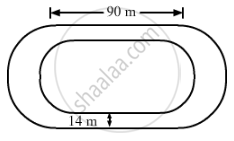Advertisement Remove all ads

# The Inside Perimeter of a Running Track Shown in the Figure is 400 M. the Length of Each of the Straight Portions is 90 M, and the Ends Are Semicircles. If the Track is 14 M Wide Everywhere, Find - Mathematics

Sum

The inside perimeter of a running track shown in the figure is 400 m. The length of each of the straight portions is 90 m, and the ends are semicircles. If the track is 14 m wide everywhere, find the area of the track. Also, find the length of the outer boundary of the track.Advertisement Remove all ads

#### Solution

Length of the inner curved portion (400 - 2 × 90) = 220 m

∴ Length of each inner curved path=220/2=110  "m"

Thus, we have:

⇒ πr = 110

⇒ 22/7"r" = 110

=> "r" = (110xx7)/22

⇒ r =35m

Inner radius = 35 m
Outer radius = (35 + 14) = 49 m
Area of track = {Area of the two rectangles [each(90 × 14)] + Area of the circular ring with R = 49 m and r = 35 m)}

=(2xx90xx14)+22/7xx[(49)^2-(35)^2]

=2520 + 22/7xx(2401-1225)

= 2520 + 22/7xx1176

= 2520 + 3696

= 6216 m

​Length of the outer boundary of the track

=(2xx90+2xx22/7xx49)

= 488 m

Therefore, the length of the outer boundary of the track is 488 m and the area of the track is 6216 sq. m.

Is there an error in this question or solution?
Advertisement Remove all ads

#### APPEARS IN

RS Aggarwal Secondary School Class 10 Maths
Chapter 18 Area of Circle, Sector and Segment
Exercise 18B | Q 60 | Page 836
Advertisement Remove all ads

#### Video TutorialsVIEW ALL 

Advertisement Remove all ads
Share
Notifications

View all notifications

Forgot password?